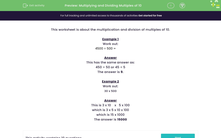# Multiply and Divide Multiples of 10

In this worksheet, students will improve their skills with multiples by multiplying and dividing multiples of 10.Key stage:  KS 3

Curriculum topic:   Number

Curriculum subtopic:   Use Four Operations for All Numbers

Difficulty level:#### Worksheet Overview

This activity is about the multiplication and division of multiples of 10.

Example 1

Work out:

4,500 ÷ 500 =

This has the same answer as:

450 ÷ 50 or 45 ÷ 5

Tip: If they both have the same number of zeros, simply remove the zeros and do the division sum as normal!

Example 2

Work out:

30 x 500

Here's the detailed explanation of what's happening:

This is 3 x 10 x  5 x 100

which is 3 x 5 x 10 x 100

which is 15 x 1,000

Here's the quick and easy way to work it out!

To work out 30 x 500:  remove the zeros, multiply 3 and 5 and then put the zeros back in again!

3 x 5 plus three zeros gives us 15,000

Does that make it easier?### What is EdPlace?

We're your National Curriculum aligned online education content provider helping each child succeed in English, maths and science from year 1 to GCSE. With an EdPlace account you’ll be able to track and measure progress, helping each child achieve their best. We build confidence and attainment by personalising each child’s learning at a level that suits them.

Get started